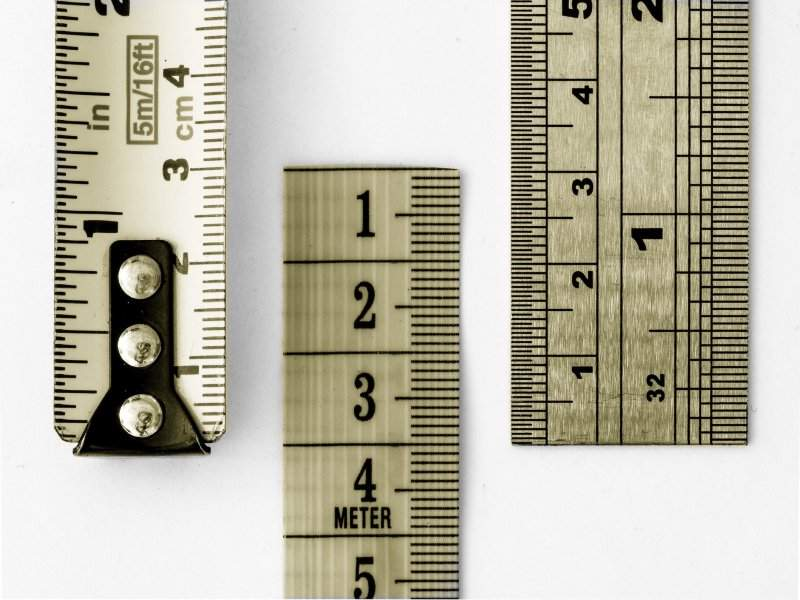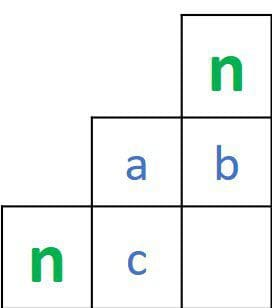# Optimizing the Levenshtein Distance for Measuring Text Similarity

For speeding up the calculation of the Levenshtein distance, this tutorial works on calculating using a vector rather than a matrix, which saves a lot of time. We’ll be coding in Java for this implementation.Photo by William Warby on Unsplash

The Levenshtein distance is a text similarity metric that measures the distance between 2 words. It has a number of applications, including text autocompletion and autocorrection.

For either of these use cases, the word entered by a user is compared to words in a dictionary to find the closest match, at which point a suggestion(s) is made. The dictionary may contain thousands of words, and thus the response of the application for comparing 2 words will likely take a few milliseconds.

The Levenshtein distance is usually calculated by preparing a matrix of size `(M+1)x(N+1)`—where M and N are the lengths of the 2 words—and looping through said matrix using 2 for loops, performing some calculations within each iteration. This makes it time-consuming to calculate the distance between a word and a dictionary of thousands of words.

For speeding up the calculation of the Levenshtein distance, this tutorial works on calculating using a vector rather than a matrix, which saves a lot of time. We’ll be coding in Java for this implementation.

The sections covered in this tutorial are as follows:

• Why use a vector rather than a matrix?
• Working with vectors
• Java implementation

### Why use a vector rather than a matrix?

First, let’s quickly summarize how the calculation of the distance works for a matrix before working with a vector.

For 2 words, such as `nice` and `niace`, a matrix of size `5x6` is created, as shown in the next figure. Note that the labels in blue are not part of the matrix and are just added for clarity. You can freely decide to make a given word represent rows or columns. In this example, the characters of the word `nice` represent the rows.Note that there are an additional row and column in the matrix that doesn’t correspond to any character in the 2 words. They are placed there to help calculate the distance.

The first row and column of the matrix are initialized by values starting at `0` and incrementing by `1` for each character. For example, the first row has values that start from 0 to 5. Note that the final distance between the 2 words is located at the bottom-right corner, but to reach it, we have to calculate the distances between all subsets in the 2 words.

Based on the initialized matrix, the distances between all subsets of the 2 words will be calculated. The process starts by comparing the first subset in the first word (which contains only 1 character ) to all subsets in the second word. Then another subset of the first word (which contains 2 characters) is compared with all subsets of the second word, and so on.

Based on the matrix in the previous figure, the first character from the word `nice` is `n`. It will be compared to all subsets of the second word `niace` —even with the subset that has zero characters `{_, n, ni, nia, niac, niace}`.

Let's see how the distance between these 2 subsets is calculated. For a given cell at the location `(i,j)` corresponding to the intersection between the 2 characters `A` and `B`, we compare the values at the 3 locations (`i,j-1`), (`i-1,j`), and (`i-1,j-1`). If the 2 characters are identical, then the value at location (`i,j`) equals the minimum of the mentioned 3 locations. Otherwise, it will be equal to the minimum value at those 3 locations after adding `1`.

Something to note here. When calculating the distances in the second row of the matrix, the cells at locations (`i-1,j`) and (`i-1,j-1`) are just indices. For the distances in the second column of the matrix, the cells at locations (`i,j-1`) and (`i-1,j-1`) are also just indices.

The previous discussion could be represented as a matrix of 4 values, where there are 3 known values and 1 missing value, as given in the next figure.Such a missing value is calculated by comparing the 3 values according to the following:

``````dist(X,Y) = min(a, b, c)      -   if X==Y
dist(X,Y) = min(a, b, c) + 1  -   if X!=Y``````

By applying that, the next figure gives the values in the second row of the matrix.After calculating the distances in the second row, the first row distances will be no longer needed. For the third row distances, we’re just using the values in the second row, not the first.

In other words, we are only in need of a single row of known values for calculating the distances for a row of unknown values—no need for an entire matrix. Using a matrix to calculate the distance saves all rows in cases where each row is used only once.

To solve that issue, we won’t use a matrix at all, but instead, a vector.

Did you know: Machine learning isn’t just happening on servers and in the cloud. It’s also being deployed to the edge. Fritz AI has the developer tools to make this transition possible.

### Working with vectors

For calculating the distance using a vector, the first step is to create that vector. The vector length will be equal to the length of a given word `+1`. If the first word `nice` is selected, then the vector length is `4+1=5`. The vector is initialized by zeros, as shown below.The zeros in that vector will be replaced by the distances between all the subsets in the selected word `nice`, and the first subset in the other word `niace`, which is just the character `n`. How do we calculate such distances?

When a matrix was used, there were 3 values to be compared, as discussed in the previous section. What is the case for a vector? There are just 2 values to be compared when calculating the distances with the first subset in the second word only.

Assuming that we’re calculating the distance for the element with index `i` and the distances vector is named `distanceVector`, then the 2 values are as follows:

1. The previously calculated distance: `distanceVector[i-1]`
2. The index of the previous element: `i-1`

The returned distance is the minimum of these 2 values:

``distanceVector[i] = min(distanceVector[i-1], i-1)``

Here’s the vector after calculating the distances between all subsets of the word `nice` and the first subset of the second word `niace`.After calculating the distances between all subsets of the first word and the first subset of the second word, we can move into calculating the distances between all subsets in the first word and the remaining subsets in the second word. The distances will be calculated by comparing the following 3 values:

1. The previously calculated distance: `distanceVector[i-1]`
2. The previously calculated distance: `distanceVector[i]`
3. The index of the element being compared to the second word: `j`

Note that `i` refers to the index of the element from the first word.

Now that we’ve clarified how the Levenshtein distance works, both with matrices and vectors, the next section implements the distance via a vector in Java.

### Java implementation

The first thing to do is to create 2 `String` variables holding the 2 words, in addition to initializing the distance vector. Here is how this is done in Java:

``````String token1 = "nice";
String token2 = "niace";
​
int[] distances = new int[token1.length() + 1];``````

After the vector is created, it will be initialized by zeros by default. The next step is to calculate the distances between all subsets in the first word `nice`, and the first subset in the second word `niace` using a `for` loop.

The next and final step is to calculate the distances between all subsets in the first word and the remaining subsets in the second word, according to the following code:

The final distance between the 2 words is saved into both the variable `dist` and the last element of the vector `distances`.

Here is a complete class named `LevenshteinDistance`, in which a method named `levenshtein()` holds the code for calculating the distance. The method accepts the 2 words as arguments and returns the distance. Inside the `main()` method, an instance of the method is created to calculate the distance between the 2 words `nice` and `niace`.

The print output from the previous code is:

``The distance is 1``

Here is another example for calculating the distance, using two different words:

``System.out.println("The distance is " + levenshteinDistance.levenshtein("congratulations", "conmgeautlatins"));``

The result is as follows:

``The distance is 5``

### Conclusion

This tutorial discussed calculating the Levenshtein distance using just a vector to return the distance between words. Being optimized to use just a vector, it could be applied for applications running on mobile devices for automatic completion and correction of words as user type searches or other textual inputs.

Bio: Ahmed Gad received his B.Sc. degree with excellent with honors in information technology from the Faculty of Computers and Information (FCI), Menoufia University, Egypt, in July 2015. For being ranked first in his faculty, he was recommended to work as a teaching assistant in one of the Egyptian institutes in 2015 and then in 2016 to work as a teaching assistant and a researcher in his faculty. His current research interests include deep learning, machine learning, artificial intelligence, digital signal processing, and computer vision.

Original. Reposted with permission.

Related:

By subscribing you accept KDnuggets Privacy Policy

By subscribing you accept KDnuggets Privacy Policy

By subscribing you accept KDnuggets Privacy Policy